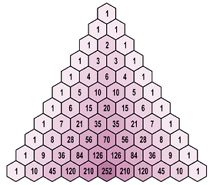Algebra

# Binomial Theorem: Level 4 Challenges

$\large \sum_{k=0}^n \left[ (-1)^k \dbinom{n}{k} (n-k)^n \right] = \ ?$$\large\displaystyle \sum_{k=1}^{504} \left ( 4k-1 \right ) \binom{2015}{4k-1}$

If the sum above can be written as $p.q^{r}$, where $p$, $q$ and $r$ are positive integers with $q$ being a prime.

Evaluate $p+q+r$.

###### Image Credit: Wikimedia TED-43.

Let a natural number $n$ be good if there exist two distinct non-integral real numbers $a$ and $b$ such that $a^k - b^k$ is an integer for all $1 \leq k \leq n$.

Find the number of natural numbers which are not good.

Compute the remainder when $\dbinom {2014}{1} + \dbinom {2014}{4} + \dbinom {2014}{7} + \cdots + \dbinom {2014}{2014}$ is divided by $1000$.

Find the number of odd coefficients in the expansion of $(a + b)^{2015}.$

×

Problem Loading...

Note Loading...

Set Loading...